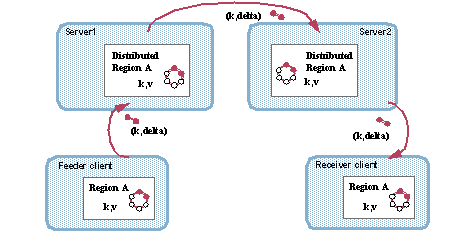Apache Geode

# Delta Propagation Example

This topic provides an example of delta propagation.

In this example, the feeder client is connected to the first server, and the receiver client is connected to the second. The servers are peers to each other.The example demonstrates the following operations:

1. In the Feeder client, the application updates the entry object and puts the entry. In response to the `put`, Geode calls `hasDelta`, which returns true, so Geode calls `toDelta` and forwards the extracted delta to the server. If `hasDelta` returned false, Geode would distribute the full entry value.
2. In Server1, Geode applies the delta to the cache, distributes the received delta to the server’s peers, and forwards it to any other clients with interest in the entry (there are no other clients to Server1 in this example)
3. In Server2, Geode applies the delta to the cache and forwards it to its interested clients, which in this case is just the Receiver client.

This example shows the basic approach to programming a `Delta` implementation.

``````package delta;

import org.apache.geode.Delta;
import org.apache.geode.InvalidDeltaException;
import java.io.DataInput;
import java.io.DataOutput;
import java.io.IOException;
import java.io.Serializable;

/**
* Sample implementation of Delta
*
* @author GemStone Systems, Inc.
* @since 6.1
*/
public class SimpleDelta implements Delta, Serializable {

// Original object fields
private int intVal;
private double doubleVal;

// Added for delta - one boolean per field to track changed status
private transient boolean intFldChd = false;
private transient boolean dblFldChd = false;

public SimpleDelta(){}

public SimpleDelta(int i, double d){
this.intVal = i;
this.doubleVal = d;
}

public boolean hasDelta() {
return this.intFldChd || this.dblFldChd;
}

public void toDelta(DataOutput out) throws IOException {
System.out.println("Extracting delta from " + this.toString());
// Write information on what has changed to the
// data stream, so fromDelta knows what it's getting
out.writeBoolean(intFldChd);
if (intFldChd) {
// Write just the changes into the data stream
out.writeInt(this.intVal);
// Once the delta information is written, reset the delta status field
this.intFldChd = false;
System.out.println(" Extracted delta from field 'intVal' = "
+ this.intVal);
}
out.writeBoolean(dblFldChd);
if (dblFldChd) {
out.writeDouble(this.doubleVal);
this.dblFldChd = false;
System.out.println(" Extracted delta from field 'doubleVal' = "
+ this.doubleVal);
}
}

public void fromDelta(DataInput in) throws IOException, InvalidDeltaException {
System.out.println("Applying delta to " + this.toString());
// For each field, read whether there is a change
// Read the change and apply it to the object
System.out.println(" Applied delta to field 'intVal' = "
+ this.intVal);
}
System.out.println(" Applied delta to field 'doubleVal' = "
+ this.doubleVal);
}
}
// In the setter methods, add setting of delta-related
// fields indicating what has changed
public void setIntVal(int anIntVal) {
this.intFldChd = true;
this.intVal = anIntVal;
}